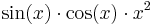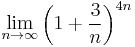# Informatics1-2019/Lab10

(Változatok közti eltérés)

# Sage

## Server

You can use this, or install it on your own from here: http://www.sagemath.org/

### Using variables

1. Let Y be your year of birth, M the month, and D the day, create these variables.
2. How much is Y divided by D? Associate this value with the b variable.
3. Let r be the remainder of Y / M.
4. What's the difference r - b?

### Symbolic calculations

1. Is it true that the square root of the square of a number is the number itself?
1. Use bool to convert to logical value
2. Is it true for real numbers? Positive numbers? (assume)
2. Prove (xy)(x + y) = x2y2
3. Prove ( − 1)2n = 1 where n is integer!

### Sage functions, methods

1. Is 2018 a prime? (use the is_prime() function)
2. Were you born on a prime day? (use the D variable!)
3. Solve the equation Dx2 + Mxbr = 0 using the solve(f, variable) function!
• x needs to be a symbolic variable!
• f can be a function, formula or an equation.
4. Solve the equation numerically! Use the find_root(f, min, max) function, where min and max defines an interval where Sage looks for the solution.
• this only works is you have one variable!
5. Solve the above equation symbolically (make D, M, b, r symbolic variables, then use solve)!
6. Differentiate the function$\sin(x)\cdot\cos(x)\cdot x^2$.
7. Integrate the previous function.
8. Calculate the limit of$\lim_{n\to\infty}\bigg(1 + \frac{3}{n}\bigg)^{4n}$
9. Let f be the following function: f(x,y) = (x+2*y)^3
10. Substitute 3 into x; then 4 into x and 2 into y. What's the result? ( use the subs() method of f)
11. Expand f! (expand())
12. Using the above, calculate the Taylor series of$\sin(x)\cdot\cos(x)\cdot x^2$ up until the 4th member. (you can differentiate and integrate a function f by f.diff(x))

### Plotting with Sage (plot)

1. Plot a cosine curve from 0 to 4*pi!
2. Plot the (x − 2)2 + 3 polynomial from -2 to 4, color it green!
3. Plot next to the previous one (using the show function) the function x3 − 3 * x + 6 in red!
4. Plot a circle: cirlce((coordinates of the center), radius, optional). The "optional" can be: color, aspect_ratio=True so that the ratio of the x and y axis are kept, otherwise we might get an ellipse.